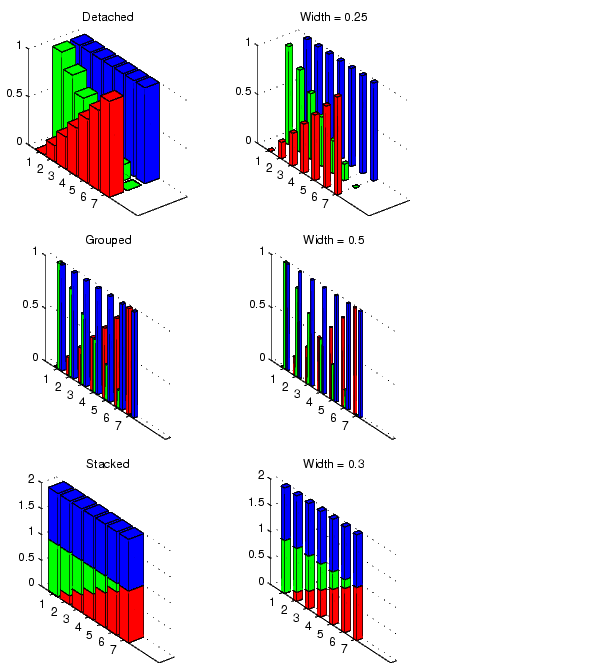MATLAB Function Referencebar3, bar3h

Three-dimensional bar chart

Syntax

• bar3(Y)
bar3(x,Y)
bar3(...,width)
bar3(...,'style')
bar3(...,LineSpec)
h = bar3(...)

bar3h(...)
h = bar3h(...)

Description

bar3 and bar3h draw three-dimensional vertical and horizontal bar charts.

bar3(Y) draws a three-dimensional bar chart, where each element in Y corresponds to one bar. When Y is a vector, the x-axis scale ranges from 1 to length(Y). When Y is a matrix, the x-axis scale ranges from 1 to size(Y,2), which is the number of columns, and the elements in each row are grouped together.

bar3(x,Y) draws a bar chart of the elements in Y at the locations specified in x, where x is a monotonic vector defining the y-axis intervals for vertical bars. If Y is a matrix, bar3 clusters elements from the same row in Y at locations corresponding to an element in x. Values of elements in each row are grouped together.

bar3(...,width) sets the width of the bars and controls the separation of bars within a group. The default width is 0.8, so if you do not specify x, bars within a group have a slight separation. If width is 1, the bars within a group touch one another.

bar3(...,'style') specifies the style of the bars. 'style' is 'detached', 'grouped', or 'stacked'. 'detached' is the default mode of display.

• 'detached' displays the elements of each row in Y as separate blocks behind one another in the x direction.
• 'grouped' displays n groups of m vertical bars, where n is the number of rows and m is the number of columns in Y. The group contains one bar per column in Y.
• 'stacked' displays one bar for each row in Y. The bar height is the sum of the elements in the row. Each bar is multi-colored, with colors corresponding to distinct elements and showing the relative contribution each row element makes to the total sum.

bar3(...,LineSpec) displays all bars using the color specified by LineSpec.

h = bar3(...) returns a vector of handles to patch graphics objects. bar3 creates one patch object per column in Y.

bar3h(...) and h = bar3h(...) create horizontal bars. Y determines the bar length. The vector x is a monotonic vector defining the y-axis intervals for horizontal bars.

Examples

This example creates six subplots showing the effects of different arguments for bar3. The data Y is a seven-by-three matrix generated using the cool colormap:

• Y = cool(7);
subplot(3,2,1)
bar3(Y,'detached')
title('Detached')

• subplot(3,2,2)
bar3(Y,0.25,'detached')
title('Width = 0.25')

• subplot(3,2,3)
bar3(Y,'grouped')
title('Grouped')

• subplot(3,2,4)
bar3(Y,0.5,'grouped')
title('Width = 0.5')

• subplot(3,2,5)
bar3(Y,'stacked')
title('Stacked')

• subplot(3,2,6)
bar3(Y,0.3,'stacked')
title('Width = 0.3')

• colormap([1 0 0;0 1 0;0 0 1])bar, barh base2dec